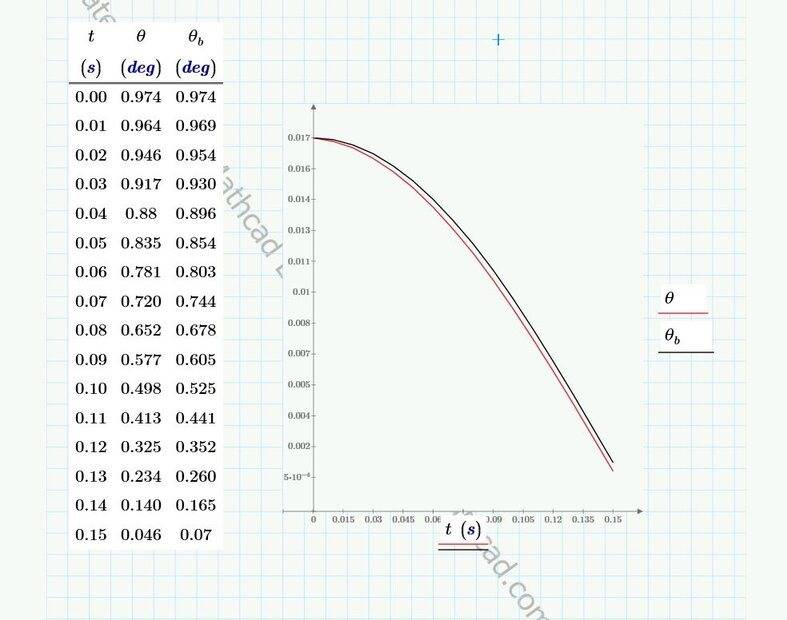# Solving for the Motion of a Spring Pendulum

• I
• erobz
In summary, the pendulum swings back and forth with a certain period. The length of the spring affects the natural period of the swing and the pendulum eventually returns to its initial state.
erobz said:
Mine was whatever was store in PTC software for 1 degree. I don't think I'm going to bother to change it. Its time consuming to manually go through all the iterations to update the table.

Fixed

View attachment 303608

Now I'm feeling much better! What do you think? Maybe I should change mine though, it looks like it will dive under yours if I do.
Why don't you just try carrying it through one complete cycle and see if it tracks. I can send you data for the rest of the cycle.

I'm doing it "manually" right now, the number of computations are getting too large for the program.

I've corrected the offset.Given that its reasonably in agreement, I probably will write the numerical solution with traditional programming. Going away for the holiday weekend though. Maybe next week?

It will be neat to see if that amount of disagreement given the potential of chaotic motion will spell disaster. Then again, when I am able to decrease my timestep perhaps they will get better.

Last edited:
•bob012345
If anyone wants to examine what produced the latest graph see the attached PDF

EDIT:
I just decreased my time step to 0.001 s for up to time 0.01 s and it is moving closer to your simulation. Thats a good sign. I'll let it at that for now.

#### Attachments

• Spring Pendulum-rev1.pdf
1.4 MB · Views: 80
Last edited:
•bob012345
I tried to program it, I think it has major theoretical issues that are tied to the assumed form of ##(2)##.

$$\ddot r + \left( \frac{k}{m} - {\dot \theta}^2 \right) r = g\cos \theta +\frac{k l_o}{m} \tag{2}$$

For the solution of ##2## to be of the form:

$$r(t) = C_1 \sin( \beta t ) + C_2 \cos ( \beta t ) + \frac{\gamma}{\beta^2}$$

the condition is:

$$\beta = \sqrt{ \frac{k}{m} - \dot{ \theta }^2 } > 0 \quad \rm{condition 1}$$

So, it is quickly failing for any sizeable angle ( non-real under the root, violating ##\rm{condition 1}## )

how about those standard numerical techniques? Any good information?

Last edited:
erobz said:
I tried to program it, I think it has major theoretical issues that are tied to the assumed form of ##(2)##.

$$\ddot r + \left( \frac{k}{m} - {\dot \theta}^2 \right) r = g\cos \theta +\frac{k l_o}{m} \tag{2}$$

For the solution of ##2## to be of the form:

$$r(t) = C_1 \sin( \beta t ) + C_2 \cos ( \beta t ) + \frac{\gamma}{\beta^2}$$

the condition is:

$$\beta = \sqrt{ \frac{k}{m} - \dot{ \theta }^2 } > 0 \quad \rm{condition 1}$$

So, it is quickly failing for any sizeable angle ( non-real under the root, violating ##\rm{condition 1}## )

how about those standard numerical techniques? Any good information?
Some basic information is here;

https://en.wikipedia.org/wiki/Numerical_methods_for_ordinary_differential_equations

But I think you really would need to put in some effort to be able to do this problem numerically from scratch. You should start with much simpler problems or the limiting cases of this problem in which the equations reduce to simpler forms. Alternatively, you can learn to use Mathematica, Matlab or some other simulator to do it for you and explore the solution space.

bob012345 said:
Some basic information is here;

https://en.wikipedia.org/wiki/Numerical_methods_for_ordinary_differential_equations

But I think you really would need to put in some effort to be able to do this problem numerically from scratch. You should start with much simpler problems or the limiting cases of this problem in which the equations reduce to simpler forms. Alternatively, you can learn to use Mathematica, Matlab or some other simulator to do it for you and explore the solution space.
Yeah, solving a coupled system of second order non-linear ODE is not covered in my textbook...

erobz said:
Yeah, solving a coupled system of second order non-linear ODE is not covered in my textbook...
I think you can turn the second order equations into first order equations of different variables.

See if this numerical treatment makes any sense for you;

•